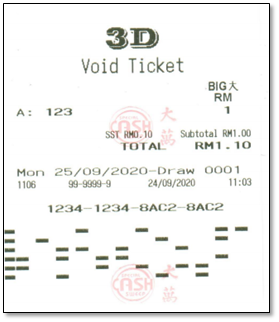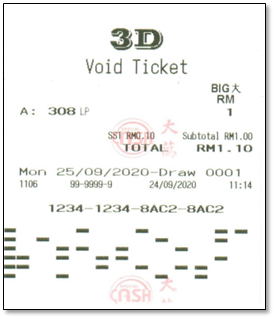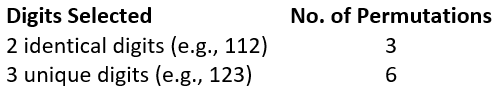Your lucky number for today 0000

# How To Play - 3D

For this game, select your 3-digit number from the 1,000 possible numbers of 000 to 999.  The 1st, 2nd and 3rd prize of the 3D Big numbers are drawn from the last 3 digits of the 1st, 2nd & 3rd prize of the 1+3D game respectively.

There are 3 ways to play:-

1.  3D Standard

Select a 3-digit number within the range of 000 to 999.2.  3D Lucky Pick

Allow the system to randomly generate a 3-digit number for you.3.  3D Permutation

Select a 3-digit number and let the system generates all possible permutations of numbers.

The total possible number of digit permutations will be dependent on the selected 3-digit number. The possibilities are :-